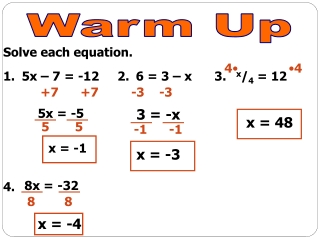DownloadDownload Presentation1. 2. 3. 4.

# 1. 2. 3. 4.

Télécharger la présentation## 1. 2. 3. 4.

- - - - - - - - - - - - - - - - - - - - - - - - - - - E N D - - - - - - - - - - - - - - - - - - - - - - - - - - -
##### Presentation Transcript

1. Warm Up Solve each equation. 4• •4 • 1. 2. 3. • 4. 5x – 7 = -12 6 = 3 – x x/4 = 12 +7 +7 -3 -3 5x = -5 3 = -x x = 48 5 5 -1 -1 x = -1 x = -3 8x = -32 8 8 x = -4

2. 6.1 Solve Linear Inequalities Objective – To be able to solve linear inequalities Standard 4.0 Students simplify expressions before solving linear equations and inequalities in one variable.

3. Inequality Signs

4. –10 –10 –10 –10 –9 –9 –9 –9 –8 –8 –8 –8 –7 –7 –7 –7 –6 –6 –6 –6 –5 –5 –5 –5 –4 –4 –4 –4 –3 –3 –3 –3 –2 –2 –2 –2 –1 –1 –1 –1 0 0 0 0 1 1 1 1 2 2 2 2 3 3 3 3 4 4 4 4 5 5 5 5 6 6 6 6 7 7 7 7 8 8 8 8 9 9 9 9 10 10 10 10 • Graph • x > 5 • x < -4 • x ≤ -1 • x ≥ 2

5. –10 –10 –9 –9 –8 –8 –7 –7 –6 –6 –5 –5 –4 –4 –3 –3 –2 –2 –1 –1 0 0 1 1 2 2 3 3 4 4 5 5 6 6 7 7 8 8 9 9 10 10 Example 2 Write an inequality represented by the graph. a) b) x ≤ 7 x > -4

6. –10 –9 –8 –7 –6 –5 –4 –3 –2 –1 0 1 2 3 4 5 6 7 8 9 10 Example 3 Solve and graph3 < m – 5 +5 +5 8 < m

7. –10 –9 –8 –7 –6 –5 –4 –3 –2 –1 0 1 2 3 4 5 6 7 8 9 10 Example 4 Solve and graph x + 8  1 -8 -8 x  -7

8. ClassworkPage 297 #12 – 26Graph Any 5 Problems You want

9. 6.2 Solve Multistep Inequalities Objective – To be able to solve multistep inequalities CA State Standard 4.0: Students simplify expressions before solving linear equations and inequalities in one variable.

10. Solving Multistep Inequalities Whenever you multiply or divide by a negative number, YOU MUST FLIP THE SIGN.